# NCERT Solutions for Class 8 Maths Chapter-2 Linear Equations in One Variable

The National Council of Educational Research and Training (NCERT) is an autonomous body of the Indian government that formulates the curricula for schools in India that are governed by the Central Board of Secondary Education (CBSE) and certain state boards. Therefore, students who will be taking the Class 10 tests administered by various boards should consult this NCERT Syllabus in order to prepare for those examinations, which in turn will assist those students to get a passing score.

You can also download the NCERT Solutions for Class 8 Maths Chapter-2 Linear Equations in One Variable to assist you in comprehensively reviewing the course material and achieving a higher score on your upcoming assessments.

Students are able to create a strong conceptual basis with the help of the NCERT Solutions PDFs available at SWC. This base plays a key part in the students’ preparation for the latter phases of the competitive examinations. We give comprehensive answers to challenges posed by NCERT in accordance with the methodology provided by CBSE. The students are able to effortlessly prepare all of the ideas that are presented in their respective courses better and more successfully, and they are even able to succeed in passing the most difficult competitive examinations, such as JEE Main, JEE Advanced, NEET, AIMS, and so on.

Your academic journey begins a new stage as you reach the eighth grade, and a new facet of learning awaits you. This is the year where pupils begin to acquire an interest in the topic at hand, and it is also the year in which you choose whether you will study business, humanity, or science in the following year (class 12).

As a consequence of this, having a solid foundation of knowledge in fields such as mathematics and physics comes highly recommended. The NCERT textbook for class 8 is the greatest book now available on the market for enhancing one’s education in the areas of mathematics and science. Read up on the theory that is presented in the book for grade 8 and answer all of the questions that are posed in the practice section of the NCERT textbook.

## NCERT Solutions for Class 8 Maths Chapter 2 – Exercises

### NCERT Solutions for Class 8 Maths Exercise 2.1

Solve the following questions.

Question 1.

x – 2 = 7

Solution :

x – 2 = 7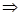x – 2 + 2 = 7 + 2X = 9

Question 2.

y + 3 = 10

Solution :

y + 3 = 10

y + 3 – 3= 10 – 3

[Subtracting 3 both sides]

= 7

Question 3.

6 = Z + 2

Solution :

6 = Z + 2

⇒ 6 – 2 = Z + 2 -2

[Subtracting 2 both sides]

⇒ 4 = z => z = 4

Question 4.

Question 5.

Question 7.

Question 9.

Question 11.

[Multiplying both sides by 3]

⇒  x = -8/5

### NCERT Solutions for Class 8 Maths Exercise 2.2

Question 1.

If you subtract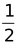from a number and multiply the result byyou get 1/8 What is the number?

Solution :

Hence, the required number is  3/4

Question 2.

The perimeter of a rectangular swimming pool is 154 m. Its length is 2 m more than twice its breadth. What are the length and breadth?

Solution :

Question 3.

The base of an isosceles triangle is 4/3cm. The perimeter of the triangle is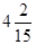cm. What is the length of either of the remaining equal sides.

Solution :

Hence, each equal side of an isosceles triangle is 7/5 cm.

Question 4.

Sum of two numbers is 95. If one exceeds the other by 15, find the numbers.

Solution :

Sum of two number = 95

Let the first number be X then another number be X + 15,.

Question 6.

Three consecutive integers add up to 51. What are these integers?

Solution :

Question 7.

The sum of three consecutive multiples of 8 is 888. Find the multiples.

Solution :

Question 8.

Three consecutive integers are such that when they are taken in increasing order and multiplied by 2, 3 and 4 respectively, they add up to 74. Find these numbers.

Solution :

Let the three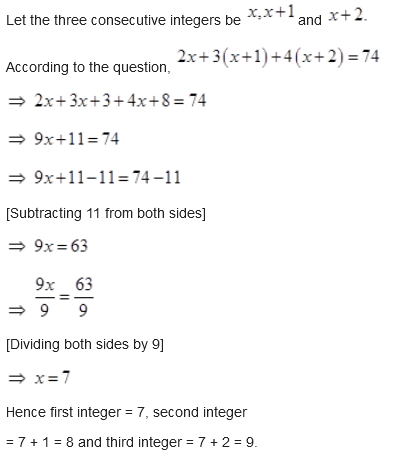.

Question 9.

The ages of Rahul and Haroon are in the ratio 5 : 7. Four years later the sum of their ages will be 56 years. What are their present ages?

Solution :

.

Question 10.

The number of boys and girls in a class are in the ratio 7 : 5. The number of boys is 8 more than the number of girls. What is the total class strength?

Solution :

Let the number of girls be x

Then, the number of boys =  x + 8

According to the question,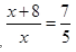[Transposing 7x to L.H.S. and 40 to R.H.S.]

[Dividing both sides by –2]

⇒ X = 20

Hence the number of girls = 20 and number of boys = 20 + 8 = 28.

Question 11.

Baichung’s father is 26 years younger than Baichung’s grandfather and 29 years older than Baichung. The sum of the ages of all the three is 135 years. What is the age of each one of them?

Solution :

Let Baichung’s age be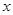years, then Baichung’s father’s age =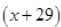years and Baichung’s granddaughter’s age =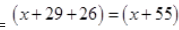years.

[Subtracting 84 from both sides]

[Dividing both sides by 3]x = 17 years

Hence, Baichung’s age = 17 years, Baichung’s father’s age = 17 + 29

= 46 years

And Baichung’s granddaughter’s age

= 17 + 29 + 26 = 72 years.

Question 12.

Fifteen years from now Ravi’s age will be four times his present age. What is Ravi’s present age?

Solution :

Let Ravi’s present age be x years.

After fifteen years, Ravi’s age = 4x  years.

Fifteen years from now, Ravi’s age = 4x years.

According to question,  4x = x + 15

⇒ 4x – x = 15

[Transposing x to L.H.S.]

[Dividing both sides by 3]

⇒ x = 5years

Hence, Ravi’s present age be 5 years.

Question 13.

A rational number is such that when you multiply it by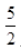and add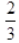to the product, you get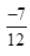What is the number?

Solution :

Let the rational number be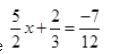According to the question,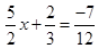[Subtractingfrom both sides]

[Dividing both sides by 60]

Hence, the rational number is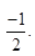Question 14.

Lakshmi is a cashier in a bank. She has currency notes of denominations 100, 50 an10 respectively. The ratio of the number of these notes is 2 : 3 : 5. The total cash with Lakshmi is 4,00,000. How many notes of each denomination does she have?

Solution :

Let number of notes be  2x,3x and 5x

According to question,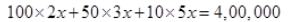[Dividing both sides by 400]

⇒ x = 1000

Hence, number of denominations of ` 100 notes =  2 × 1000 = 2000

Number of denominations of ` 50 notes =2 × 1000 = 3000

Number of denominations of ` 10 notes = 5  × 1000 = 5000

Therefore, required denominations of notes of  100,  50 and  10 are 2000, 3000 and 5000 respectively.

Question 15.

I have a total of `300 in coins of denomination 1, 2 and 5. The number of 2 coins is 3 times the number of `5 coins. The total number of coins is 160. How many coins of each denomination are with me?

Solution :

Total sum of money = 300

Let the number of 5 coins be x ,  number of 2 coins be 3x  and number of  1 coins be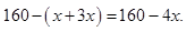According to question,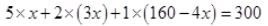[Subtracting 160 from both sides]

[Dividing both sides by 7]

⇒ x = 20

Hence, the number of coins of ` 5 denomination = 20

Number of coins of ` 2 denomination = 3 × 20= 60

Number of coins of ` 1 denomination =  160 – 4 × 20 = 160 – 80 = 80

Question 16.

The organizers of an essay competition decide that a winner in the competition gets a prize of 100 and a participant who does not win, gets a prize of 25. The total prize money distributed is `3,000. Find the number of participants is 63.

Solution :

Total sum of money =  3000

Let the number of winners of 100 be  x

And those who are not winners = 63 – x

According to the question,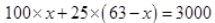Hence the number of winner is 19.

Question :2

Question :3

### NCERT Solutions for Class 8 Maths Exercise 2.4

Question 1.

5/2 from it. She multiplies the result by 8. The result now obtained is 3 times the same number she thought of. What is the number?

Solution :

Let Amina think a number x.

According to the question,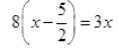Hence, the number is 4

Question 2.

A positive number is 5 times another number. If 21 is added to both the numbers, then one of the new numbers becomes twice the other new number. What are the numbers?

Ans. Let another number be x.

Then positive number = 5x.

According to the question,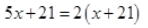Hence another number = 7 and positive number = 7 × 5 =35

Question 3.

Sum of the digits of a two-digit number is 9. When we interchange the digits, it is found that the resulting new number is greater than the original number by 27. What is the two-digit number?

Solution :

x.

Therefore, the tens place digit =  9 – x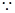2-digit number = 10 x tens place digit + unit place digit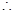Original number =  10(9 – x) + x

According to the question, New number

= Original number + 27

Hence, the 2-digit number =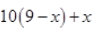= 10 (9 – 6) + 6 =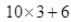= 30 + 6 = 36

Question 4.

One of the two digits of a two-digit number is three times the other digit. If you interchange the digits of this two-digit number and add the resulting number to the original number, you get 88. What is the original number?

Solution :

Let the unit place digit of a two-digit number be x.

Therefore, the tens place digit = 3x.2-digit number = 10 x tens place digit + unit place digitOriginal number =  10 × 3x  + x = 30x + x = 31x

According to the question, New number + Original number = 88

⇒ 10x + 3x  + 31x = 88

⇒ 44x = 88

⇒ x = 88/44 = 2

= 31  × 2 = 62

Question 5.

Shobo’s mother’s present age is six times Shobo’s present age. Shobo’s age five years from now will be one third of his mother’s present age. What are their present age?

Solution :

Let Shobo’s present age be x  years.

And Shobo’s mother’s present age = 6x years

According to the question,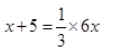Hence, Shobo’s present age = 5 years

And Shobo’s mother’s present age =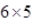= 30 years.

Question 6.

There is a narrow rectangular plot, reserved for a school, in Mahuli village. The length and breadth of the plot are in the ratio 11 : 4. At the rate `100 per meter it will cost the village panchayat`75,000 to fence the plot. What are the dimensions of the plot?

Solution :

Let the length and breadth of the rectangular plot be 11x  and 4x respectively.Perimeter of the plot =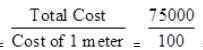= 750 m

We know that Perimeter of rectangle = 2 (length + breadth)

Therefore, according to the question,

Hence, length of rectangular plot = 11 × 25 = 275 m

And breadth of rectangular plot = 4 × 25 = 100 m

Question 7.

Hasan buys two kinds of cloth materials for school uniforms, shirt material that costs him `50 per meter and trouser material that costs him `90 per meter.

Solution :

Let ratio between shirt material and trouser material be  3x : 2x

The cost of shirt material =The selling price at 12% gain =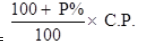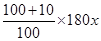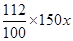=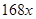The cost of trouser material =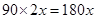The selling price at 12% gain =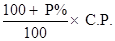= 200 meters

Hence, Hasan bought 200 meters of the trouser material.

For every 2 meters of the trouser material he buys 3 meters of the shirt material. He sells the materials at 12% and 10% respectively. His total sale is `36,000. How much trouser material did he buy?

Question 8.

Half of a herd of deer are grazing in the field and three fourths of the remaining are playing nearby. The rest 9 are drinking water from the pond. Find the number of deer in the herd.

Solution :

Let the total number of deer in the herd be x

According to question,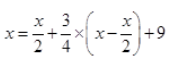Hence, the total number of deer in the herd is 72.

Question 9.

A grandfather is ten times older than his granddaughter. He is also 54 years older than her. Find their present ages.

Solution :

Let present age of granddaughter be x years.

Therefore, Grandfather’s age = 10x years

According to question,

⇒ 10x – x = 54

⇒ 9x = 54

⇒ x = 54/9 = 6  years

Hence, granddaughter’s age = 6 years and grandfather’s age = 10 × 6 = 60 years.

Question 10.

Aman’s age is three times his son’s age. Ten years ago he was five times his son’s age. Find their present ages.

Solution :

Let the present age of Amon’s son be x years.

Therefore, Aman’s age = 3x years

According to question,

### NCERT Solutions for Class 8 Maths Exercise 2.5

Solve the following linear equations.

Question 1.

Solution :

L.C.M of 2,5,3, and 4 is 60

Thus, multiplying by 60 on both the sides, we get,

Question 2.

Solution :

L.C.M of 2,6, and 4 is 12

Thus, multiplying by 12 on both the sides, we get,

Question 3.

Solution :

L.C.M of 3,6, and 2 is 6

Thus, multiplying by 6 on both the sides, we get,

Question 4.

Solution :

L.C.M of 3 and 5 is 15

Thus, multiplying by 15 on both the sides, we get,

Question 5.

Solution :

L.C.M of 4 and 3 is 12

Thus, multiplying by 12 on both the sides, we get,

Solution :

L.C.M of 2 and 3 is 6

Thus, multiplying by 6 on both the sides, we get,

Simplify and solve the following linear equations.

Question 7.

Solution :

Opening the brackets, we write,

9 =10t + 5

-9 – 5 = 10t-3t

-14 = 7t

t = -2

Question 8.

Solution :

Opening the brackets, we write,

15y – 60 – 2y + 18 + 5y + 30 = 0

18y -12 =0< /p>

18 y= 12

Question 9.

Solution : Opening the brackets, we write,

15z – 21 – 18z + 22 = 32z -52 -17

-3z + 1 = 32z – 69

– 35z = -70

Z = 2

Question 10.

0.25(4f – 3) = 0.05(10f – 9)

Solution :

Rewriting 0.25 as 1/4 and 0.05 as 1/20

### NCERT Solutions for Class 8 Maths Exercise 2.6

Solve the following equations.

Question 1.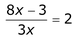‘

Solution :

Question 2.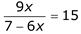Solution :

Multiplying by (7-6x) on both the sides we get,

9x = 15 (7-6x)

9x = 105 – 90x

9x + 90 x = 105

99 x = 105

Question 3.

Solution :

Multiplying by 9(z+15) on both the sides we get,

9z = 4 (z+15)

9z = 4z + 60

5z = 6

z = 12,

Question 4.

Solution :

Multiplying by 5(2-6y) on both the sides we get,

5(3y+4) = -2 (2-6y)

15y + 20 = -4 + 12y

3y = -24

y = -8

Question 5.

Solution :

Multiplying by 3(y+2) on both the sides we get,

3(7y+4) = – 4 (y+2)

21y + 12 = – 4y – 8

25y = -20

Question 6.

The ages of Hari and Harry are in the ratio 5:7. Four years from now the ratio of their ages will be 3:4. Find their present ages.

Solution :

Let the present ages of Hari and Harry are 5x and 7x

Then, four years later,  Hari age will be 5x+4 years and Harry age will be 7x + 4 years

Now

Thus, presently, Hari age is 20 and Harry age is 28

Question 7.

The denominator of a rational number is greater than its numerator by 8. If the numerator is increased by 17 and the denominator is decreased by 1, the number obtained is  3/2, Find the rational number.

Solution :

Let the numerator of rational number is x and the denominator or rational number is y.

The, Denominator = x+ 8

The rational number is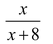Now,

## Conclusions of NCERT Solutions for Class 8 Maths Chapter-2 Linear Equations in One Variable

The academic team of SWC has provided NCERT answers for class 8 mathematics chapter 2. We’ve given solutions for all of Chapter 2’s exercises. These are step-by-step answers to all of the problems in Chapter 2 of the NCERT textbook. Read the first theoretical chapter. Make sure you’ve gone through the theoretical section of chapter 2 in the NCERT textbook and that you know the formula for the chapter.